• If you are citizen of an European Union member nation, you may not use this service unless you are at least 16 years old.

View

# Geometry

last edited by 5 years, 8 months ago

The Shapes Song

Shapes

Mathmaticious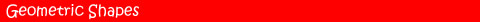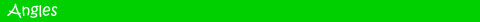Learn about geometric and 3D shapes with this fun shape game for kids. Complete a number of interactive activities involving triangles, quadrilaterals and a whole range of interesting shapes.

 Follow the onscreen instructions and learn about angles with this interactive activity for kids. Squirt various objects by rotating the water hose, how many of the targets you can hit? Give it a go and find out!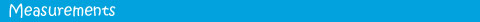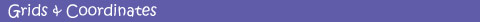Enjoy a number of fun activities related to measuring length and weight. Use the interactive scales and ruler to help measure parcels with this great educational geometry game for kids.

 Pass your driving test with this cool math game that focuses on grids and coordinates. Use the compass to move your car in a number of directions, aim for your destination and be careful not to bump into anything.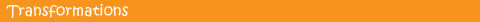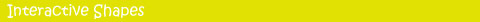This transformation game for kids helps teach the concepts of reflection, rotation and translation with fun, interactive challenges that kids will enjoy. Give it a try, see how many challenges you can complete and have fun learning math online!

 Check out this interactive shapes activity and have fun learning about geometry. Use your problem solving skills to drag and rotate different sized tiles that will fill in the missing pieces of the main shape.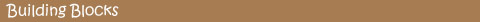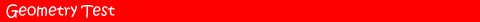Enjoy this educational building blocks game and have fun completing various shapes. Move shapes into the correct positions, earn points and move onto more difficult levels of this cool geometry game.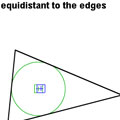Visual Geometry Test Try these visual geometry challenges that will test your spatial awareness. Have fun making accurate parallelograms, bisecting angles, judging halfway points, finding the center of circles and using your eyes to gauge accuracy.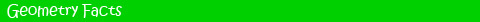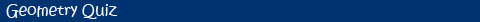Learn more about geometry with our wide range of facts. Enjoy interesting information related to circles, polygons, polyhedrons, curved 3D shapes, the history of geometry and much more.

 Put your skills to the test with our printable geometry quiz. Answer a range of questions related to geometry topics or check out our shape quiz if you want to focus on that side of geometry.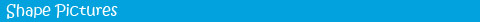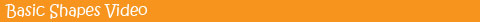Check out our great range of shape pictures and images. Find pictures of everything from simple 2D shapes to stars, quadrilaterals, prisms, pyramids, complex 3D shapes and more.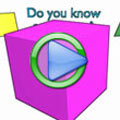Basic Shapes Video for Kids Have fun learning about basic shapes with this fun video for kids that features important shapes such as circles, triangles, hexagons and squares. How many of the shapes do you know? Check out the video and find out!

The following geometry games are suitable for elementary and middle school students.

2D Shapes (Jeopardy Game)

Classify angles as acute, right, obtuse, or straight when you play this interactive Tic Tac Toe game against the computer.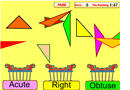Classifying Triangles Game

In this interactive game, kids will practice classifying triangles as as acute, right, or obtuse by dragging and dropping different images in the correct basket in less than two minutes.2D Shapes Game (Concentration)
In this game students click on two cards to match the figure of a two-dimensional shape with its name. If there is a match, the problems remain on the page; if not, the cards are turned over.

Classify geometrical figures as 2-dimensional or 3-dimensional by playing this fun and interactive geometry game.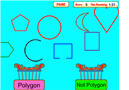Polygon or Not?

Do you know if a given geometric figure is a polygon or not? Play this fun game to demonstrate your skills! How many points can you score in one and a half minutes?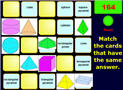3D Shapes Game (Concentration)
Have fun matching pictures of three-dimensional shapes with the correct words. If there is a match, the problems remain on the page; if not, the cards are turned over.Types of Polygons

In this game you have to quickly name different types of polygons based on given clues. For each question you will have only 30 seconds to write your answer.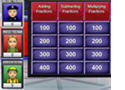Angles Jeopardy Game is a fun way to assess your knowledge about measuring and classifying angles. The game has a single-player mode and a multi-player feature.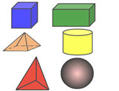3d Shapes Game

Discover the names of the most important 3d shapes.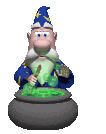Pythagorean Theorem Game

Find the legs or the hypotenuse of a right triangle, and solve word problems by applying the Pythagorean Theorem.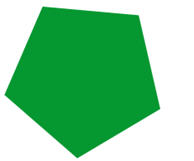Polygon Game

Learn how to clasify different polygons based on their characteristics.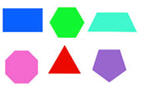Basic Shapes Game

Match six basic shapes with the correct words.

Geometry Games
Gamequarium Math Home

3D Shapes

Junior High/video

Mathmaticious

http://gogeometry.com/videos/mathmaticious_a_mathematical_parody.htm

How Round is Your Circle? Math meets Engineering

http://gogeometry.com/geometry/reuleaux_rotor_geometry.htm

Teaching Geometry and Donald in Mathmagic Land

http://gogeometry.com/videos/math_education_elearning_1.htm

http://gogeometry.com/videos/math_education_elearning_2.htm

http://gogeometry.com/videos/math_education_elearning_3.htm

Tutorials

http://www.learner.org/courses/learningmath/geometry/index.html

The following common geometry formulas are very useful when solving problems about two-dimensional figures. In the table below “b” stands for base, “h” means height, “L” stands for length, “w” represents the width, “r” is the radius of a circle, and “d” represents the diameter.When solving problems about area, perimeter, or circumference, first you need to identify the important elements in your figure. Then, find the formula that applies to your problem, and simply replace the letters with the correct values. Make the necessary computations, and don’t forget to add the unit at the end.

You can also find the area of a figure by counting the number of square units inside it (if it shows in the picture). When finding the area of a triangle (or parallelogram), you can use the length of any side as the base. The height is always perpendicular to the base.

Free Self Help Geometry Videos

http://www.tutor-usa.com/blog/index.php/free-geometry-self-help-video-tutors/

#### Geometry Nets  http://www.kidzone.ws/math/geometry/nets/

A "Geometry Net" is a flattened  out three dimensional solid (a three dimensional shape) -- like a cube, a prism or a pyramid.  When you cut out the "net", fold it and glue it together you can see what the three dimensional shape looks like.

A three dimensional shape is called three dimensional (or 3D) because it has three dimensions:  length, width and height.

The two most common measurements we need for a three dimensional shape is:

• volume:  for example, how much water it would hold
• surface area:  for example, how much area you would have to paint to cover the entire outside of the shape

Creating paper models like these geometry nets allows children to explore the more familiar 2 dimensional shapes that go into making a three dimensional one.

I think it's particularly fun to do these during Christmas -- you can hang the three dimensional shapes on the Christmas tree.

By Shape - Platonic Solids (all the sides are identical):

Geometry Net Cubes-- all the sides are squares

Geometry Net Tetrahedrons-- all the sides are triangles

By Shape - Prisms (a 3D shape where if you sliced it like a loaf of bread, each slice would look identical):

Geometry Net Rectangular Prisms-- all the slices are rectangles

Geometry Net Square Prisms-- all the slices are squares